Posts

Showing posts from May, 2021

Go Language - Program to find the duplicate words in a string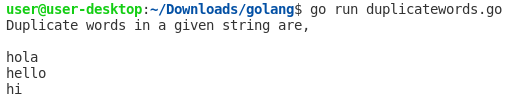Hello everyone, today we need to find out the duplicate words present in the string and print those words. Example 1: Program to find the duplicate words in a given string //Program to find the duplicate words in a string package main import ( "fmt" "strings" ) // Main function func main() { str := "hola hello hi hola good sleep hello hi night morning" //Converts the string into lowercase strl := strings.ToLower(str) //Split the string into words words := strings.Split(strl, " " ) fmt.Println( "Duplicate words in a given string are, \n" ) for i := 0 ; i < len(words); i++ { count := 1 for j := i + 1 ; j < len(words); j++ { if words[i] == words[j] { count++ //Set words[j] to 0 to avoid printing visited word words[j] = "0" } } //Displays the duplicate word if count is greater tha

Go Language - Maps - Example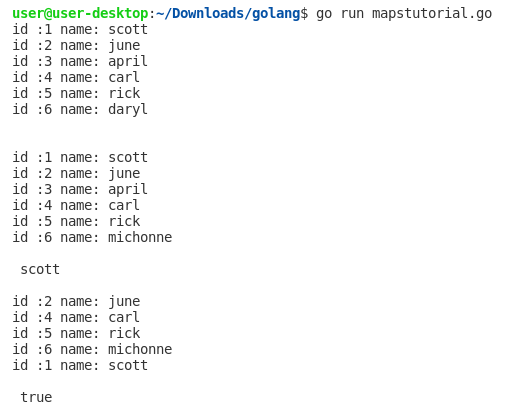A map is an unordered collection of key/value pairs, where each key is unique. We can create a new map with a make statement or a map literal. The default zero value of a map is nil. The len function returns the size of a map. Go Range keyword is used in different kinds of data structures in order to iterate over elements. Create a Map: Create a Map called users that will store int keys and String values: /* Create a new map * Empty map of string-string pairs */ users := make( map [ int ] string ) Add Items: Add a new key-value pair, //Add Items users[ 1 ] = "scott" users[ 2 ] = "june" users[ 3 ] = "april" users[ 4 ] = "carl" users[ 5 ] = "rick" users[ 6 ] = "daryl" Update Value: // Update value users[ 6 ] = "michonne" Iterate over all keys and values: // Iterating over all keys and values for id, name := range users { fmt.Printf( "id :%d

Go Arrays - Program to Find Largest & Smallest Element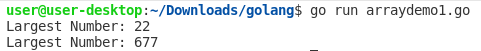Example 1: Finding the largest element in an Array // Go program to find largest number in an Array package main import "fmt" // Main function func main() { // Let’s define an array first arr :=  int { 2 , 11 , 22 , 5 , 6 , 3 , 1 , 9 } fmt.Println( "Largest Number:" , LargestNumber(arr)) //Another Example anotherarr :=  int { 144 , 666 , 99 , 677 , 433 , 422 , 255 } fmt.Println( "Largest Number:" , LargestNumber(anotherarr)) } func LargestNumber(arr  int ) int { var temp int for i := 0 ; i < len(arr); i++ { for j := i + 1 ; j < len(arr); j++ { if arr[i] > arr[j] { temp = arr[i] arr[i] = arr[j] arr[j] = temp } } } return arr[len(arr)- 1 ] } Output: Example 2: Finding the smallest element in an Array // Go program to find smallest number in an Array package main import "fmt" // Main function f

How to iterate over a map in Go?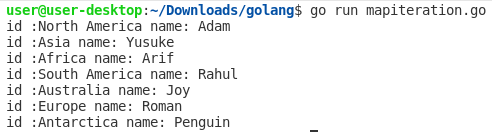A map is an unordered collection of key/value pairs, where each key is unique. Go Range keyword is used in different kinds of data structures in order to iterate over elements. Example 1: Iterate over all keys and values // Go program to illustrate how // to iterate the map package main import "fmt" // Main function func main() { // Let’s define a map first usermap := map [ string ] string { "North America" : "Adam" , "Asia" : "Yusuke" , "Africa" : "Arif" , "South America" : "Rahul" , "Australia" : "Joy" , "Europe" : "Roman" , "Antarctica" : "Penguin" , } // Iterating over all keys and values for id, name := range usermap { fmt.Printf( "id :%s name: %s\n" , id, name) } } Output: Example 2: Iterate over all k

Hashing in Go Language - MD5 ,SHA-1, SHA-256, & SHA-512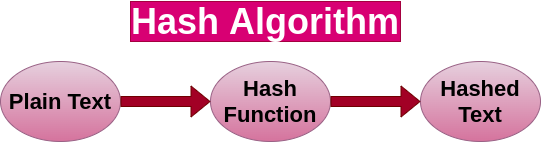What does Hashing mean? A secure password hash is an encrypted sequence of characters obtained after applying certain algorithms and manipulations on user-provided password, which are generally very weak and easy to guess. Please remember that once this password hash is generated and stored in the database, you can not convert it back to the original password. MD5 hash in Go The MD5 message-digest algorithm is a widely used hash function producing a 128-bit hash value. Although MD5 was initially designed to be used as a cryptographic hash function, it has been found to suffer from extensive vulnerabilities. It can still be used as a checksum to verify data integrity, but only against unintentional corruption. It remains suitable for other non-cryptographic purposes, for example for determining the partition for a particular key in a partitioned database. Example: Go-MD5 to hash a String package main //Required imports import ( "crypto/md5" "encoding/hex"

Go Language - RSA Encryption And Decryption PKCS Example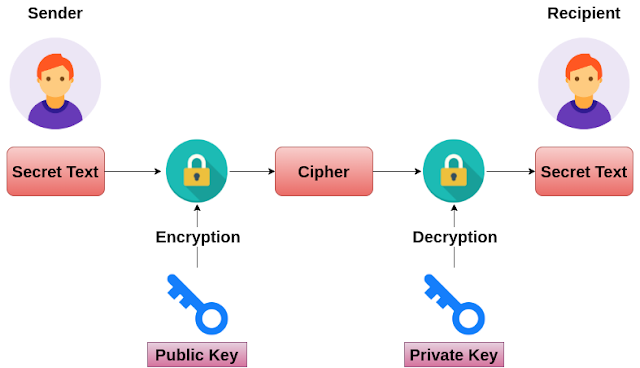The RSA algorithm (Rivest-Shamir-Adleman) is a cryptographic algorithm that is used for specific security services or purposes, which enables public-key encryption and is widely used to secure sensitive data, particularly when it is being sent over an insecure network such as the HTTP. A public key is shared publicly, while a private key is secret and must not be shared with anyone. The following illustration highlights how asymmetric cryptography works: RSA Encryption and Decryption with PKCS1v15 Example, package main //Required imports for Encryption & Decryption import ( "crypto/rand" "crypto/rsa" "encoding/base64" "fmt" ) func main() { //2048 is the number of bits for RSA bitSize := 2048 //Generate RSA keys privateKey, err := rsa. GenerateKey(rand.Reader, bitSize) if err != nil { panic(err) } publicKey := privateKey.PublicKey //Your secret text secretMessage := "

Go - Palindrome Program Example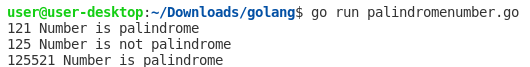If a number/string, which when read in both forward and backward way is the same, then such a number is called a palindrome number. Example 1. Go Program to check whether the given number is Palindrome or not //Check Whether a Number is Palindrome or Not package main import "fmt" // Main function func main() { number1 := 121 number2 := 125 number3 := 125521 IsPalindrome(number1) IsPalindrome(number2) IsPalindrome(number3) } func IsPalindrome(number int ) { var lastDigit int sum := 0 a := number // Code to reverse a number for a != 0 { lastDigit = a % 10 //getting remainder sum = (sum * 10 ) + lastDigit a = a / 10 } /*If given number equal to sum than number is palindrome otherwise not palindrome*/ if sum == number { fmt.Println(number, " is palindrome " ) } else { fmt.Println(number, " is not palindrome" ) } } Output: Example 2. Go Progra